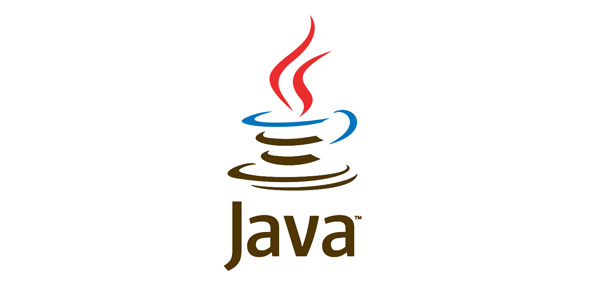# Itemp Java Quiz 5

18 Questions | Total Attempts: 253SettingsChapter 5 Revision

• 1.
public class Converter {           public static void main(String args [ ]) {                    Converter con = new Converter();                    con.dollartoRupees(15);          }           double dollartoRupees(int dollar) {                     System.out.println (dollar+" dollar is equivalent to Rs" +dollar*25);            } } What will be the output of this program?
• A.

Dollar is equivalent to Rs 375

• B.

15 dollar is equivalent to Rs 375

• C.

Dollar dollar is equivalent to Rs 375

• D.

This program gives a run time error

• 2.
Arguments to methods always appear within __________.
• A.

Square brackets [ ]

• B.

Parentheses ( )

• C.

Curly braces { }

• D.

Quotation marks " "

• 3.
Suppose your method does not return any value, which of the following keywords can be used as return type?
• A.

Void

• B.

Int

• C.

Double

• D.

Public

• 4.
An object is an instance of a __________.
• A.

Program

• B.

Class

• C.

Method

• D.

Data

• 5.
The keyword __________ is required to declare a class.
• A.

Public

• B.

Private

• C.

Class

• D.

All the above

• 6.
Analyze the following code: class Test {    public static void main(String[] args){               System.out.println(findNumber(5));       }      public static int findNumber(int n) {               System.out.println("int");               return n;        }        public static long findNumber(long n) {                 System.out.println("long");                 return n;         } } What will happen when you execute this block of code?
• A.

The program displays int followed by 5.

• B.

The program displays long followed by 5.

• C.

The program runs fine but displays things other than given in (A) and (B).

• D.

The program does not compile because the compiler cannot distinguish which findNumber to invoke.

• 7.
All Java applications must have a method called __________.
• A.

Main()

• B.

Init()

• C.

Main()

• D.

Init()

• 8.
A method can be declared with no parameters. TRUE or FALSE?
• A.

True

• B.

False

• 9.
You must have a return statement in a non-void method. TRUE or FALSE?
• A.

True

• B.

False

• 10.
You can declare two variables with the same name in __________.
• A.

A method

• B.

A block

• C.

Two nested blocks in a method

• D.

Different methods in a class

• 11.
Variables that are shared by every instances of a class are __________.
• A.

Public variables

• B.

Private variables

• C.

Instance variables

• D.

Class variables

• 12.
A variable that is associated with an individual object is called __________.
• A.

A static variable

• B.

A class variable

• C.

An instance variable

• D.

An object reference variable

• 13.
When you write more than one method with the same name but different parameters in the same class, then it is called __________.
• A.

• B.

Override

• C.

Copy

• D.

Function

• 14.
What modifier should you use on a class so that a class in the same package can access it but a class in a different package cannot access it?
• A.

Public

• B.

Private

• C.

Protected

• D.

Use the default modifier.

• 15.
When a new key word is used to create an instance of a class,
• A.

Java allocates memory for the object.

• B.

Initialises the instance variables.

• C.

Calls the constructor.

• D.

All the above

• 16.
• A.

True

• B.

False

• 17.
A constructor always has a return type.
• A.

True

• B.

False

• 18.
The this key word is used to
• A.

Declare a variable

• B.

Initialize a variable

• C.

Refer to the current object

• D.

Create an object

Related TopicsBack to top